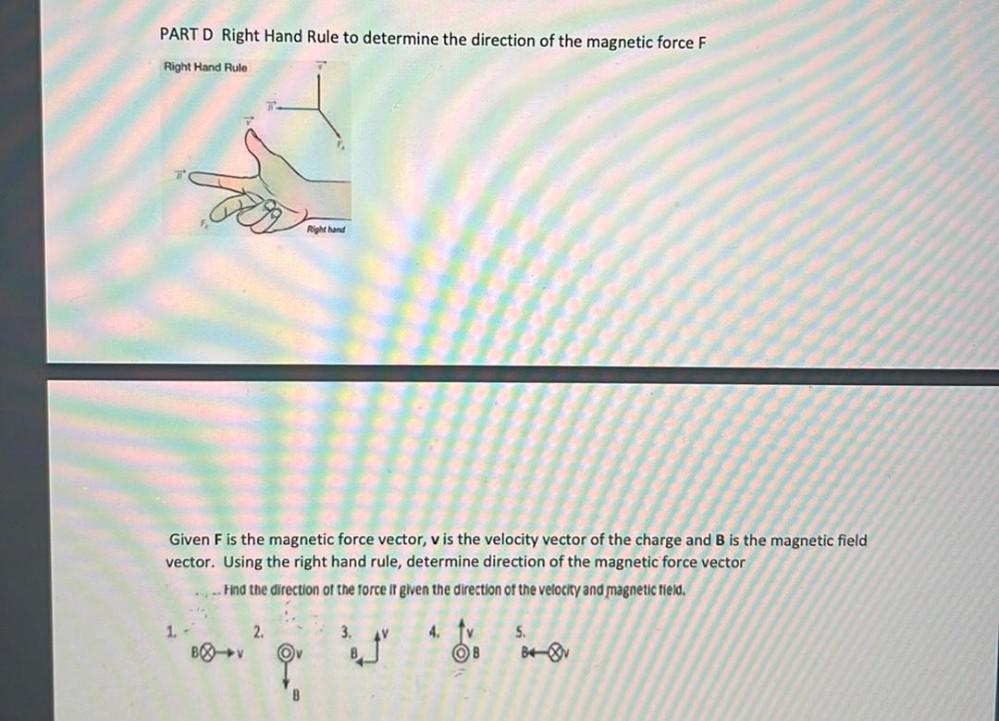Question:

# PART D Right Hand Rule to determine the direction of the magnetic force F Right Hand Rule Right hand Given F is the magnetic forPART D Right Hand Rule to determine the direction of the magnetic force F Right Hand Rule Right hand Given F is the magnetic force vector, v is the velocity vector of the charge and B is the magnetic field vector. Using the right hand rule, determine direction of the magnetic force vector .: -- Find the direction of the force it given the direction of the velocity and magnetic field. 1 2. 3. 4. BOY to 5. BV Ov B B